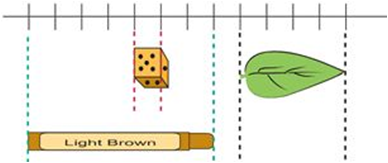# Indian Talent Olympiad - NMITO PDF Sample Papers for Class 3

Resources:

Class 3 sample paper & practice questions for National Mathematics Indian Talent Olympiad (NMITO) level 1 are given below. Syllabus for level 1 is also mentioned for these exams. You can refer these sample paper & quiz for preparing for the exam.Q.1 Q.2 Q.3 Q.4 Q.5 Q.6 Q.7 Q.8 Q.9 Q.10
 Q.1 Which of the following statements is false?  (IMO L2 2016) a) The sum of two odd numbers is an odd number. b) The difference between two even numbers is an even number. c) The difference between two odd numbers is an even number. d) The sum of an odd number and an even number is an odd number.
 Q.2 How many sevens are there in 735? (IMO L2 2012) a) 15 b) 105 c) 17 d) 150
 Q.3 Arrange these numbers from the greatest to the smallest:56, 3 × 8, 29, 2 × 7, 2, 19.   (IMO L2 2012) a) 56, 2 × 7, 3 × 8, 29, 2, 19. b) 2, 2 × 7, 19, 3 × 8, 29, 56. c) 56, 29, 3 × 8, 19, 2 × 7, 2. d) 2 × 7, 3 × 8, 29, 2, 19, 56.
 Q.4 Choose the correct option: The die is 1 cm from every side, then  the leaf is _________ cm long.a) 10 cm b) 4 cm c) 6 cm d) 8 cm
 Q.5 Kala goes to the temple every day at quarter to eleven. What time does she go to the temple? a) 10:45 b) 11:15 c) 11:45 d) 11:30
 Q.6 Choose the short form of the following:8000 + 200 + 50 + 6 a) 82.56 b) 8.256 c) 0.8256 d) 8,256
 Q.7 The sum of two numbers is 98,000 and one number is 42,361. Find the other number: a) 65,639 b) 51,342 c) 55,639 d) 50639
 Q.8 A field is in the form of a rectangle, 30 metres long and 20 metres wide. What is the total sum of its length and breadths? a) 50 m b) 100 m c) 30 m d) 120 m
 Q.9 Which of the two fractions are equivalent? a) 5/2 and 2/5 b) 1/4 and 2/4 c) 4/3 and 8/6 d) 2/3 and 1/3
 Q.10 What are the missing numbers in the boxes?738/6 = (____)/ (___) a) 122, 4 b) 102, 1 c) 123, 1 d) 122, 5Sample PDF of Indian Talent Olympiad - National Mathematics Indian Talent Olympiad (NMITO) PDF Sample Papers for Class 3: